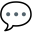We could not find any results for:

### ToplistsIt looks like you aren't logged in.

Subscribe Login/RegisterRegister to chat with like-minded investors on our interactive forums.

# FTSE 100: Mixed Signals

Print

Sometimes the analysis is not strong enough to suggest the market will move up or down. We are in this situation with regard to the FTSE 100.There are two ways to interpret the Elliott wave pattern, either the FTSE is in the midst of a decline to 7400 or it is at the start of a rally to 8000. Take a look at this chart:The decline since May is a correction in three waves [W,X,Y] which is wave (B). The question is about wave (B), did this move end at 7477 which is the recent low?

To answer this question we need to identifies where wave X ended. If wave X was the rally in three waves [a,b,c (circle)] ending at 7790, wave (B) is not yet complete. In this scenario wave a (circle) was the rising wedge in July. This is because we are in wave Y and this wave will be in three waves [a,b,c (circle)]. If wave X ended at 7790 the low at 7477 is the bottom of wave a (circle) inside wave Y, the current rebound is wave b (circle). The next move is wave c (circle) to 7400.

In the alternate wave count on the chart below, we have a bullish scenario if we assume that wave X ended at 7783:In this scenario wave X was the rising wedge ending at 7783, wave Y is an expanded flat [a,b,c (circle)] ending at 7477. In the expanded flat pattern wave b (circle) will end above the top of wave a (circle) which is what we have here. This means wave (B) is complete and the FTSE will rally.

Because of the conflicting signals there is not enough evidence to suggest buying the FTSE at current levels, unless you trade intraday.